# 沃罗诺伊纹理着色器节点¶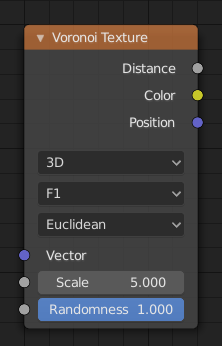## 输入选项¶

Texture coordinate to evaluate the noise at; defaults to Generated texture coordinates if the socket is left unconnected.

W

Texture coordinate to evaluate the noise at.

Scale of the noise.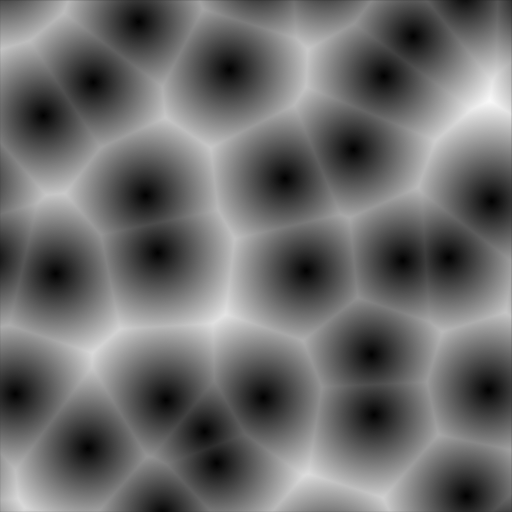平滑度: 0.0.¶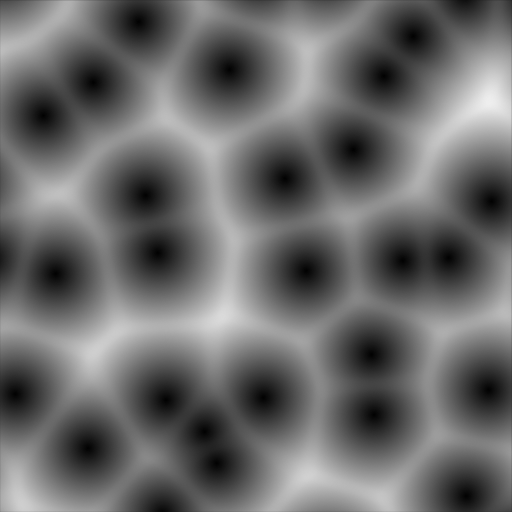平滑度: 0.25.¶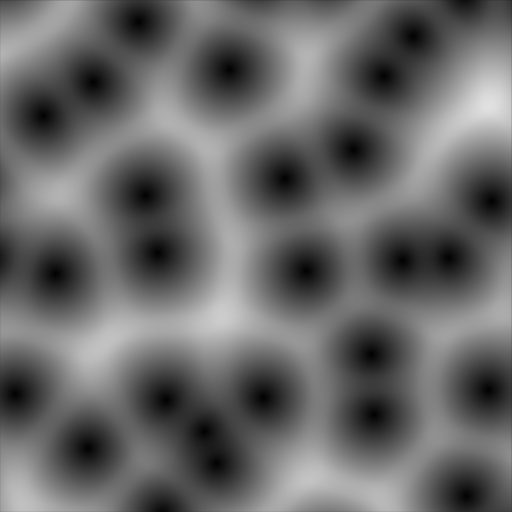平滑度: 0.5.¶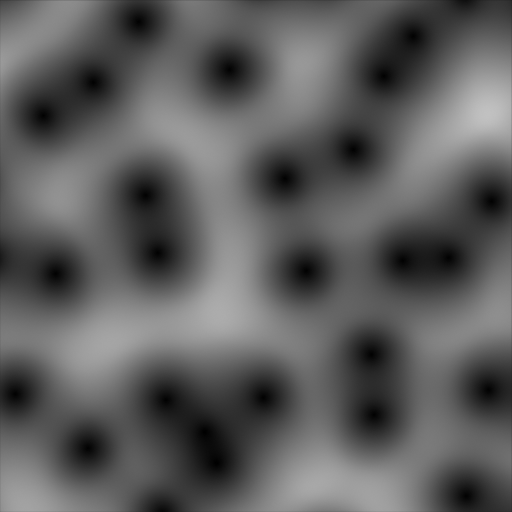平滑度: 1.0.¶平滑度: 0.0.¶平滑度: 0.25.¶平滑度: 0.5.¶平滑度: 1.0.¶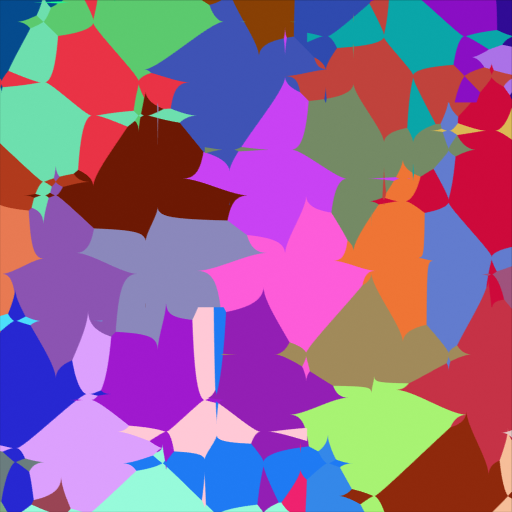指数: 0.5.¶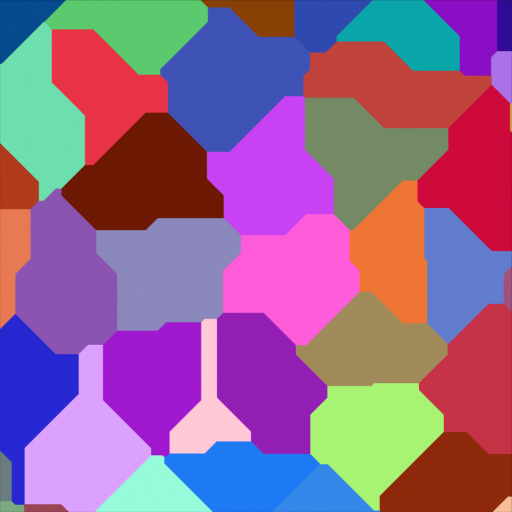指数: 1.0.¶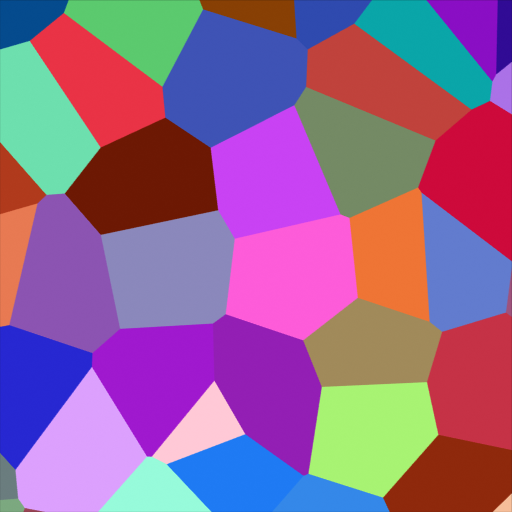指数: 2.0.¶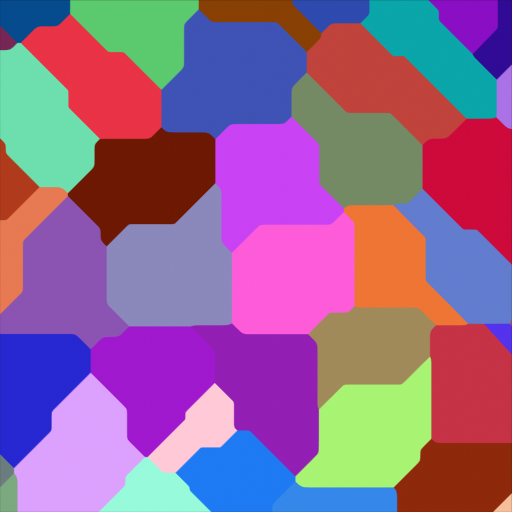指数: 32.0.¶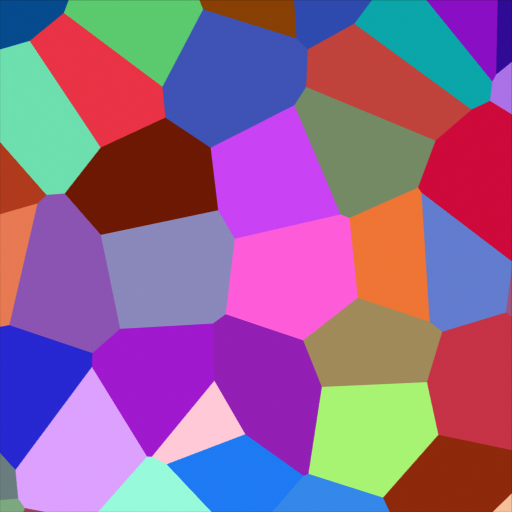随机性: 1.0.¶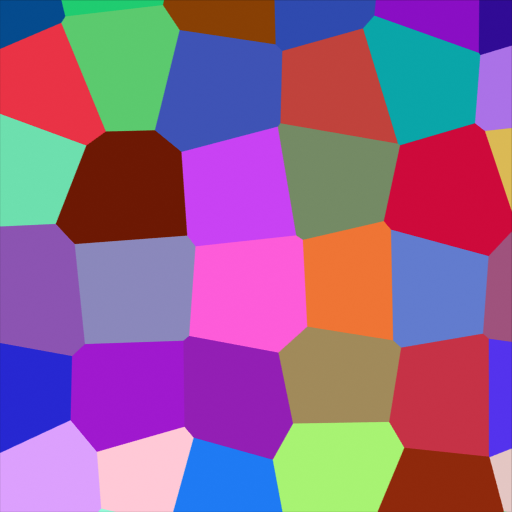Randomness: 0.5.¶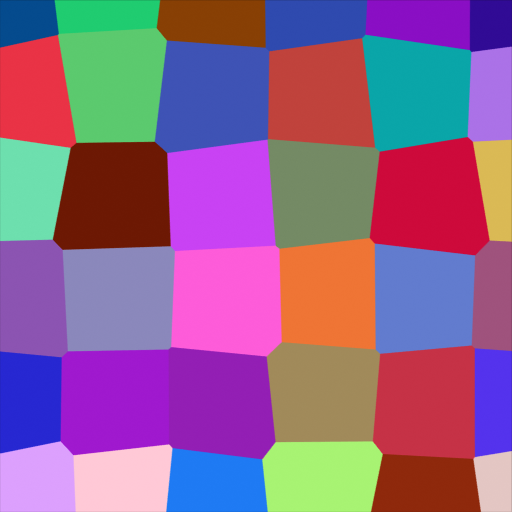Randomness: 0.25.¶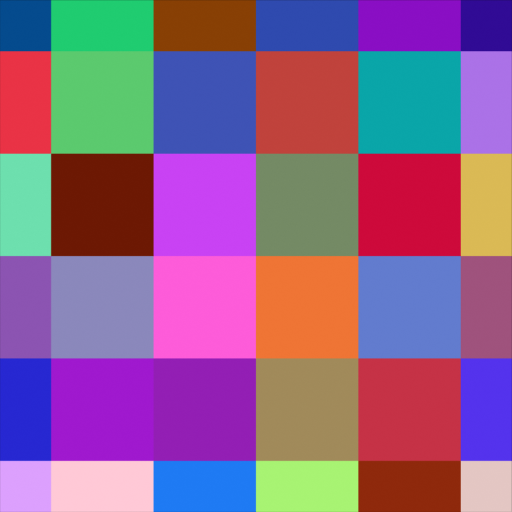Randomness: 0.0.¶

## 属性¶

1D

Evaluate the noise in 1D space at the input W.

2D

Evaluate the noise in 2D space at the input Vector. The Z component is ignored.

3D

Evaluate the noise in 3D space at the input Vector.

4D

Evaluate the noise in 4D space at the input Vector and the input W as the fourth dimension.

Higher dimensions corresponds to higher render time, so lower dimensions should be used unless higher dimensions are necessary.

The Voronoi feature that the node will compute and return.

F1

Compute and return the distance to the closest feature point as well as its position and color.距离。¶颜色。¶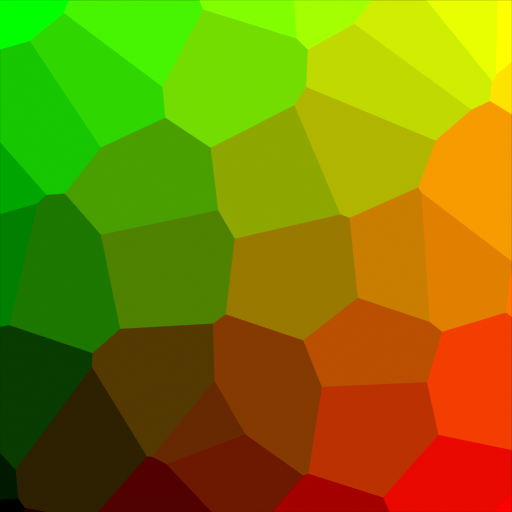位置。¶
F2

Compute and return the distance to the second closest feature point as well as its position and color.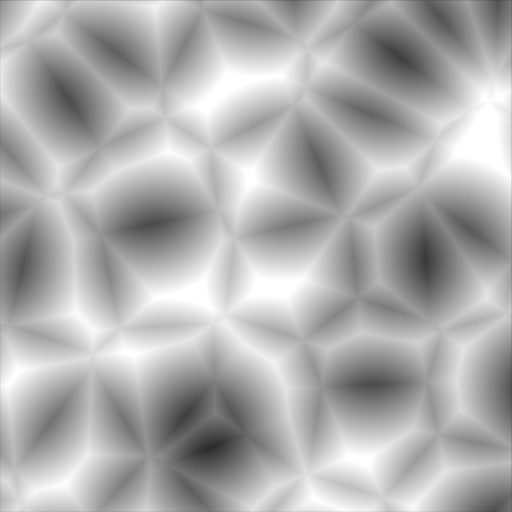距离。¶颜色。¶位置。¶

Compute and return a smooth version of F1.距离。¶颜色。¶位置。¶
Distance to Edge

Compute and return the distance to the edges of the Voronoi cells.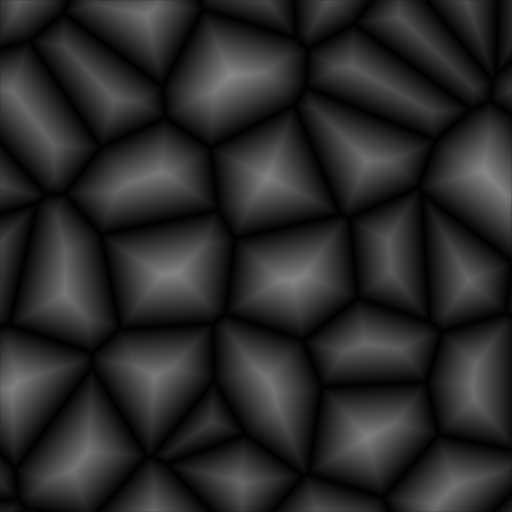距离。¶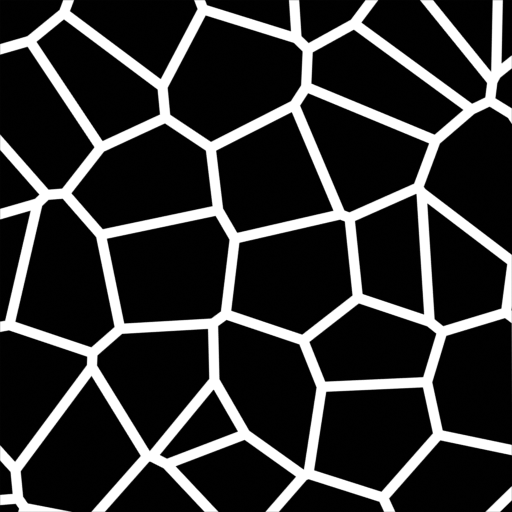距离 < 0.05。¶
N 球体半径

Compute and return the radius of the n-sphere inscribed in the Voronoi cells. In other words, it is half the distance between the closest feature point and the feature point closest to it.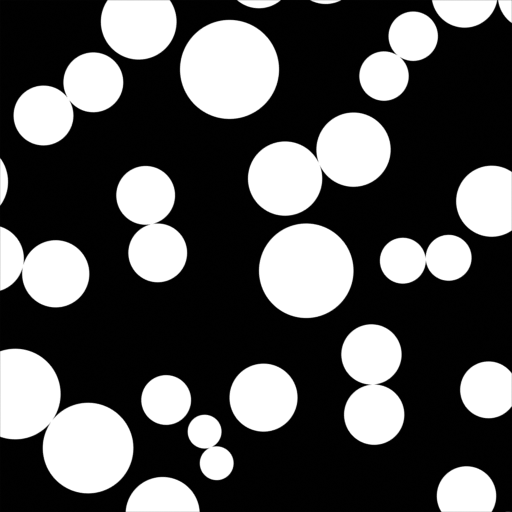The n-sphere radius can be used to create tightly packed n-spheres.¶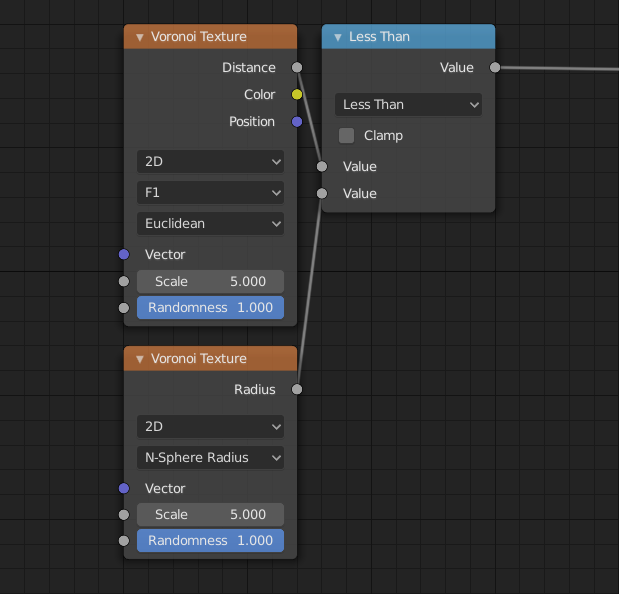Node tree for the shader to the left.¶

The distance metric used to compute the texture.

Use the Euclidean distance metric.

Use the Manhattan distance metric.

Use the Chebychev distance metric.

Use the Minkowski distance metric. The Minkowski distance is a generalization of the aforementioned metrics with an Exponent as a parameter. Minkowski with an exponent of one is equivalent to the Manhattan distance metric. Minkowski with an exponent of two is equivalent to the Euclidean distance metric. Minkowski with an infinite exponent is equivalent to the Chebychev distance metric.闵可夫斯基指数: 0.5(闵可夫斯基 1/2)。¶闵可夫斯基指数: 1.0(曼哈顿)。¶闵可夫斯基指数: 2.0(欧几里得)。¶闵可夫斯基指数: 32.0(切比雪夫近似)。¶

W

N维球面半径。

## 举例¶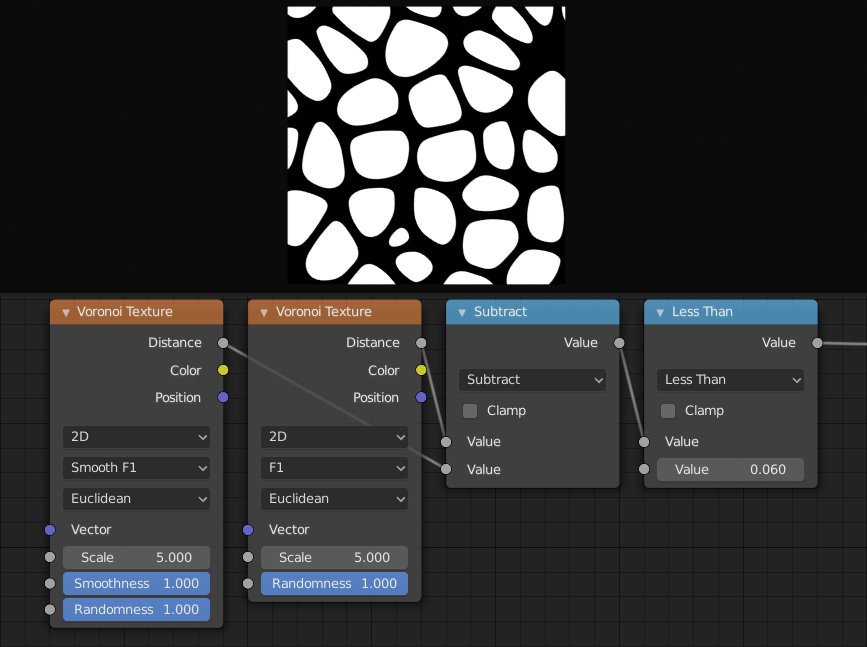The difference between F1 and Smooth F1 can be used to create beveled Voronoi cells.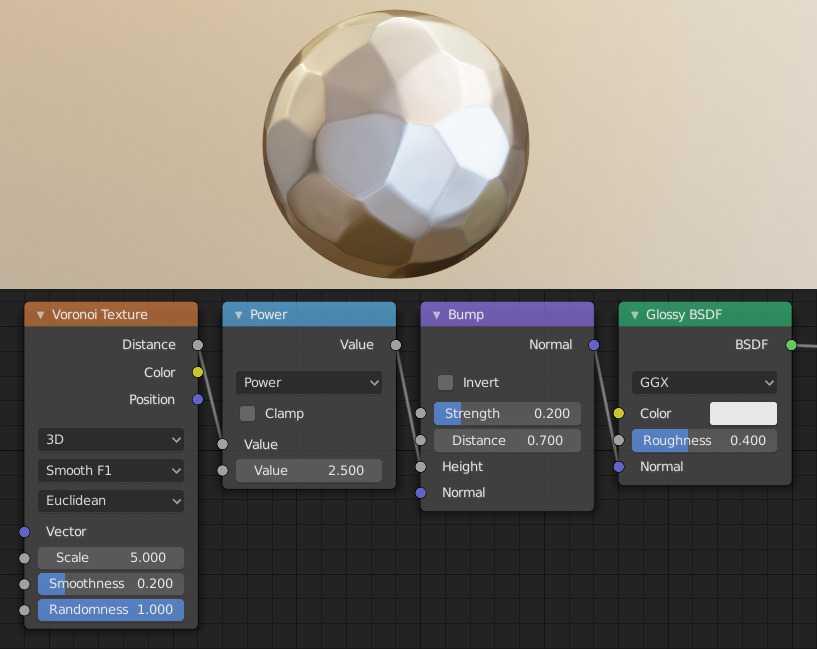Creating a hammered metal shader using the Voronoi Texture node.